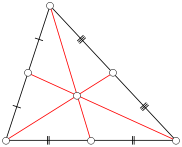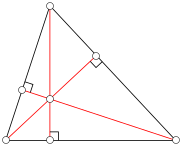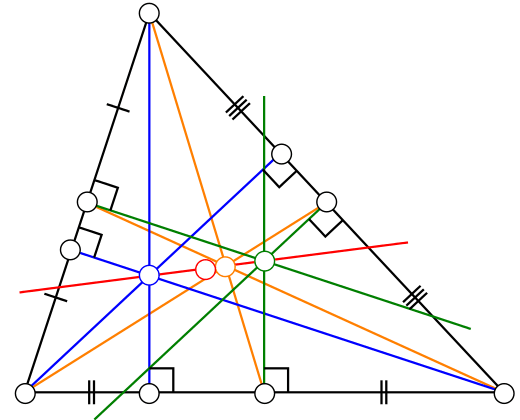Well, I probably saw it as a student years ago, and apparently it’s standard, but I came across this today through Olympiad wanderings and thought it was wonderful enough to share.

It’s a fun fact from Euclidean geometry — the sort of gem which in a decent world would be taught in an advanced secondary school maths class. But of course it is not, at least nowhere near me — and indeed even the basic ideas on which it relies (including almost all geometry and indeed the very notion of proof) have more or less been ruthlessly exterminated from the high school curriculum. As is happening now, with the content of the Australian school mathematics curriculum being shifted further back and, so it seems, further watered down, in the name of teaching abstract problem solving skills… skills so abstract that there is less and less actual concrete knowledge to apply them to.

And while not taught in schools, this sort of thing is also too “elementary” to appear in most university courses — unless the university has a specialised “elementary” mathematics course, perhaps aimed at future mathematics teachers, that might cover it. Even then the subject matter can’t be taught by those future teachers, as the content lies in a different universe from that where the curriculum lives, a superlunary realm inaccessible from such a small base of material.

It is just triangle geometry, in some sense no more than a clever elaboration of similar triangles and similar ideas. So it falls through the cracks, only to be learned by those precocious enough to be taught it in an Olympiad programme, or already interested enough to read Euclidean geometry for their own interest.

Anyway, the fact here is a fact about triangles. I’ll try not to get too snarky about the curriculum.

Triangles in the plane. Just the nice, flat, Euclidean, plane.

It’s a fact about centres of triangles. Triangles have centres.

But not one, oh no, no one. They have many centres. There is a whole world of triangle centres out there, none of which you are likely ever to see in any curriculum any time soon, being too far from mundane everyday applications. (Imagine if just one of these was included in a curriculum! Ah, we can dream. Imagine the problems you could solve, the proofs and abstractions and explorations you could do, if you even knew what these things were. Which you don’t.)

So yes, as it turns out there are many ways in which you might define the “centre” of a triangle. Here are three of the most standard ones. Let the triangle’s vertices be $$A,B,C$$, so its sides are $$AB, BC$$ and $$CA$$.

• The centroid. It’s often called G. This is what you get when you join each vertex to the midpoint of the opposite side. Those three lines, called medians, meet at this point G, called the centroid. It’s also the centre of mass of the triangle. (Oh what, a practical application? Who would have thought.)• The circumcentre. It’s often called O. As it turns out, there is a unique circle which passes through the three vertices A,B,C. A basic fact, again, which is not in any curriculum anywhere near me. This circle is called the circumcircle of ABC. (Well, it circumnavigates the vertices, doesn’t it?) Its centre O is called the circumcentre.• The orthocentre. It’s often called H. This is what you get when you drop a perpendicular from each vertex to the opposite side. Those three lines, called altitudes (because they measure the height of the vertex above each side), also meet at a point H. That point H is called the orthocentre.(All diagrams public domain from wikipedia.)

Now, it’s not even clear that any of these points exist. (No, the pictures do not constitute proofs.) If you take three lines in the plane, they may or may not meet at a point. Usually they won’t. But in special cases they will. As it turns out, the three medians of a triangle are sufficiently special that they do meet at a point. As are the three altitudes.

Most likely any reader here will either know about all three of these centres, or about none of them. If you know all three, congratulations my friend. If not, let me simply say this about them:

• The fact a centre of mass of a triangle exists shouldn’t be too surprising. And a median splits a triangle into two halves of equal area (same height, half of the base each), so it shouldn’t be too surprising that the centre of mass should lie on a median, hence on all the medians, so that it seems they at least ought to meet at a point. In fact, if you know vectors, then if we take an origin $$O$$ and position vectors $$\vec{OA}, \vec{OB}, \vec{OC}$$ for A,B,C, then the position vector of the centroid G is their average, $\vec{OG} = \frac{ \vec{OA} + \vec{OB} + \vec{OC} }{3}$.
• The fact that you can put a circle through three points shouldn’t be too surprising. Given two points, you can draw many many circles through them, in fact so many that they can pass through any other point as well. Or, for a more misanthropic take on the matter, have you ever tried to keep equally away from 2 people? Well, I suppose it depends on how obnoxious or irritating are the people near you or wherever you happen to go, but in any case it’s not that hard, you just have to stay on a line bisecting those two people (it’s called a perpendicular bisector). Well, now take it a step further and suppose there are 3 people. It can be done. When you do that you intersect another perpendicular bisector and at their intersection, in fact all three perpendiculars have to meet, and that point is the same distance from all the people. You are now at the circumcentre and you can put a circle through all those who repel you. (Well, you’d likely feel trapped between all 3, and you’d probably end up running away, but then you won’t be equally far away from the repellent ones. Anyway…)
• The fact that the orthocentre is much less obvious, at least to me, without using some other mathematical theorems. It is a bit surprising, without knowing mathematics. But no matter, because I’ll prove it as part of the gem I’m about to explain.

It’s not just the orthocentre. Mathematics is full of surprising, counterintuitive, even apparently ridiculous ideas and statements that turn out to be true. And the process of turning them from apparently ridiculous to understood — then even intuitive — ideas, is the idea of proof. Which you will never learn in a mathematics curriculum any time soon, except in the very uppermost advanced stream in the last year or two, if you’re lucky. Without the language of proof, there will be no words in which to express this process.

So, the centroid $$G$$, the circumcentre $$O$$, the orthocentre $$H$$. Three very different but equally reasonable claimants to the title of *centre* of a circle. They are not the same. But as it turns out, amazingly, they always lie on a line. This was proved by Leonhard Euler in 1767, and the line is called the Euler line of the triangle. Drawing everything on one diagram results in a suitably impressive picture of this line, and hopefully gives some impression of how surprising it is. (Source: wikipedia.)There are many proofs of this fact. Many of them are very nice, using some combination of similar triangles, or transformation geometry. And as with all proofs of facts that are initially surprising, it converts a possibly surprising, possibly ridiculous statement into an incontrovertible, natural, and true one.

The proof I’m going to share uses vectors. So to understand it, you need to know something about vectors: including position vectors of points; addition, subtraction and scalar multiplication of vectors; and the dot product, and the fact that the dot product of two nonzero vectors is zero if and only if they’re perpendicular. If you don’t, oh well, go and learn it. You likely won’t be able to derive it yourself, even with your mad 21st century skillz.

Remember, $$G$$ is the centroid, $$O$$ is the circumcentre and $$H$$ is the orthocentre. But I’m not even going to assume we even know the orthocentre exists yet.

Let’s set up some position vectors. We’ll take the circumcentre $$O$$ as the origin. (After all, we often use $$O$$ for the origin and $$O$$ for the circumcentre. Why not both? Quite a convenient elision.) As $$O$$ is the centre of a circle passing through $$A,B,C$$ we have $$| \vec{OA} | = | \vec{OB} | = | \vec{OC} |$$. And, as I mentioned above, as $$G$$ is the centre of mass of the triangle, $$\vec{OG} = ( \vec{OA}+\vec{OB}+\vec{OC})/3$$.

We’re now ready to state a theorem.

Theorem: Let $$H$$ be the point with position vector $$\vec{OH} = \vec{OA}+\vec{OB}+\vec{OC}$$. Then the orthocentre of $$ABC$$ exists and is equal to $$H$$, and $$\vec{OH} = 3 \vec{OG}$$.

This theorem tells us a lot. Firstly, that the orthocentre exists, i.e. that the three altitudes of ABC intersect. Secondly, that they intersect at this point $$H$$ defined by $$\vec{OH} = \vec{OA}+\vec{OB}+\vec{OC}$$. Thirdly, from $$\vec{OH} = 3 \vec{OG}$$, recalling how scalar multiplication works, it tells us that $$O,H,G$$ all lie on a line, with $$O, G, H$$ in order along it, and with $$H$$ 3 times as far away from $$O$$ as $$G$$.

In fact, the conclusion that $$\vec{OH} = 3 \vec{OG}$$ isn’t really much of a conclusion. It is immediate from the way we defined $$H$$. We defined $$H$$ by $$\vec{OH} = \vec{OA}+\vec{OB}+\vec{OC}$$. But since the centroid G satisfies $$\vec{OG}=( \vec{OA}+\vec{OB}+\vec{OC})/3$$, we observe that $$\vec{OH}$$ is just 3 times $$\vec{OG}$$. Of course 1 is 3 times 1/3…

So the thing to prove is that the orthocentre exists and is equal to $$H$$, defined in this way. The proof is very short. And that’s what I mean when I say “one line Euler line”.

Proof: We show $$H$$ lies on the altitude from $$A$$ to $$BC$$, i.e. that $$AH$$ is perpendicular to $$BC$$: $\vec{AH}.\vec{BC} = (\vec{OH}-\vec{OA}).(\vec{OB}-\vec{OC}) = (\vec{OB}+\vec{OC}).(\vec{OB}-\vec{OC}) = |\vec{OB}|^2 – |\vec{OC}|^2 = 0.$ A similar argument shows that $$H$$ lies on the other altitudes of $$ABC$$, hence the altitudes intersect at $$H$$.
QED

By “one line” I mean the one line calculation above. (If indeed it fit on one line on your screen…) Let’s explain each step. First, $$\vec{AH} = \vec{OH}-\vec{OA}$$; that’s how vector subtraction works. Second, we substituted $$\vec{OH} = \vec{OA}+\vec{OB}+\vec{OC}$$, so that $$\vec{OH} – \vec{OA} = \vec{OB} + \vec{OC}$$. Third, we expanded a dot product “difference of perfect squares”, and used the fact that the dot product of a vector with itself is the length squared of that vector. Finally, we used the fact that $$O$$ is the circumcentre, so that $$|\vec{OB}|=|\vec{OC}|$$.

If you’d like to make sure you understand this, try to do to the “similar” arguments that $$H$$ lies on the other altitudes of $$ABC$$. In other words, as an exercise, try to show that $$\vec{BH}.\vec{AC} = 0$$ and $$\vec{CH}.\vec{AB} = 0$$.

Well, as I say, this is all in some sense “standard knowledge”, just stuff which is inaccessible to pretty much every normal person since nothing remotely in the same universe is ever taught at school (and nor will it be any time soon anywhere near me). As I say I just came across and (re-)discovered it, and thought of it was worth sharing.

The fact that $$\vec{OH} =\vec{OA}+\vec{OB}+\vec{OC}$$ is apparently known as Sylvester’s triangle problem, and what I’ve shown here is very similar to it; in essence it’s the same thing. This vector proof isn’t new, it appears in many places online and off. And you can find many other more standard type proofs, using similar triangles and such things, online and off. As for proving the existence of the orthocentre, cut the knot has a nice page of different proofs, including one along the lines above.

And there you have it. A one line Euler line. More or less. So to speak.

I would dearly love it if students could solve problems related to these ideas — or even much simpler versions thereof —  without knowing any geometry or the idea of proof beyond the pale shadow of which exists in our curriculum, simply by the application of “21st century problem solving skills”. Futuristic indeed they must be to derive everything from a few flawed lessons on proof and some drilling of ways to prove triangles congruent. But as it turns out, to do this sort of problem solving, the average person needs to learn some geometry first. You cannot expect the average student derive hundreds of years of mathematical progress and geometric ingenuity by themselves. With the words to say and the musical notes to play, you can sing your song and create your mathematical world. Once you have the ideas sorted out, you open up a whole universe of creativity, problem solving, a route to the stars, and you have a basis for learning 21st century problem solving skills of creativity, abstraction, precision, rationality, logic, powered by passion, insight, and intuition. But without them, what can you do but flail about. “Every year fewer and fewer words, and the range of consciousness always a little smaller.

Wouldn’t it be nice if ideas like these, or at an attenuated version, or at least something approximating them — or just something, something? — could at least make an appearance in our schools?

One line Euler line
Tagged on: